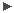# Common Trending and QC tools: Documentation

tqs = Trending and Quality Control Systemmake printable new: see also: v1.4: - supports KPI plots (interprets config file section 4.7 with REF_VALUES) all trendPlotter config files trendPlotter tool trendPlotter operations matplotlib documentation here topics: COMPUTE and FIT rules | axis labels | installation

# The python engine for trendPlotter: PET

Purpose

PET is the Python Engine for trendPlotter. Since summer 2012 it replaces the MIDAS plot engine which was used in trendPlotter before version 3.0. It has been developed by Burkhard Wolff.

It is not a stand-alone tool but works exclusively as plugin to trendPlotter. Therefore the following documentation does not cover operational issue but only configuration details, in particular related to the syntax for the COMPUTE and FIT rules.

Syntax changes from MIDAS to python:COMPUTE and FIT rules

Compute and fit rules (identifies by COMPUTE_RULE and FIT_RULE in the config.tp_ files) have to be rewritten into Python syntax. Old MIDAS syntax is ignored; the plot will be created but the result will not look as desired, and an email warning will be sent by the tool. (In the following, the term RULES is used for COMPUTE_RULEs and FIT_RULES).

Any Python statement that can be written in one line of code can be used to describe the RULES. If a statement cannot be comfortably written into one line, use a file to store the statements and refer to it with the execfile command (see below).

The x, y, and time values for each parameter set can always be accessed via the names xdat, ydat, and tdat.

Some examples are presented here. If necessary, a 'translation' of the python code is added as last line (the documentation of all matplotlib commands is available here). In some cases, the statements extend over two lines for better readability. In the actual configuration, only one line must be used.

 Multiplying the y values of the data points by 1000 MIDAS COMPUTE_RULE CONV2 &&comp/tab plt_ch2.tbl #2 = #2*1000.0&& PYTHON COMPUTE_RULE CONV2 &&ydat = 1000.0*ydat&&

 Correct zeropoints from ADUs to electrons MIDAS COMPUTE_RULE CORR_Q1 &&comp/tab new3Q1.tbl :zeropoint = :zeropoint + 2.5 * log10(1.75)&& PYTHON COMPUTE_RULE CORR_Q1 &&ydat = ydat + 2.5 * math.log10(1.75)&&

 Plot a linear function (x values from 0 to 60000, slope 0.0157, black colour, dotted line) MIDAS FIT_RULE FITLINQ1 &&cre/ima linQ1 1,60 0,1000 POLY 0,1 ; comp/ima linQ1 = 0.0157 * linQ1 ; over/row linQ1 ? ? ? 2&& PYTHON FIT_RULE FITLINQ1 &&pylab.plot([0,60000],[0,0.0157*60000],'k:')&& <> E.g. in GIRAFFE DETNOISE plot.

 Plot a square root MIDAS COMPUTE_RULE FITSQRTQ1 &&cre/ima rootQ1 1,60 0,1000 POLY 0,1 ; comp/ima rootQ1 = sqrt(rootQ1/1.75) ; over/row rootQ1 ? ? ? 2&& PYTHON COMPUTE_RULE FITSQRTQ1 &&xvals=numpy.arange(0,60000,1000,dtype=numpy.float32); yvals=numpy.sqrt(xvals/1.75); pylab.plot(xvals,yvals,'k:')&& <> E.g. in GIRAFFE DETNOISE plot.

 Plot an additional vertical line to mark an MJD value: PYTHON FIT_RULE MJDMARK &&pylab.plot([55814,55814],[0,0.22],'b-')&& <> E.g. in many WISQ plots.

One can also use multi-valued Python lists to simultaneously plot many lines on a trending plot. For example, to mark horizontal lines indicating detectors number 65, 69, 73, 77, 81, 85, 89, and 93 one can do the following:

 plot many horizontal lines in one go: PYTHON FIT_RULE FIT_LINES &&hline = [65, 69, 73, 77, 81, 85, 89, 93]; pylab.plot([0,60000],[hline, hline], 'k:')&& <>

Labels

 Plot an additional label in relative figure coordinates (from 0 to 1): PYTHON FIT_RULE LAB1 &&pylab.figtext(0.1,0.9,'fixed-pattern noise',color='r')&& <> Plot a label with the current median of the data values: LAB2 &&pylab.figtext(0.1,0.9,'median:'+str('%5.2f'%average)+'min',color='r')&& or: &&pylab.figtext(0.1,0.9,'median: %5.2f min'%average,color='r')&& <> This trick is used in the WISQ exectimes plots .

 Plot an additional label in data coordinates: PYTHON FIT_RULE LAB2 &&pylab.text(50000,120,'photon noise',color='g')&& <>

External files and programmes
If there are many plotting statements (more than can easily be accommodated in one line), one might collect them in an external file and execute it as Python code (it's a good idea to give it the extension .py and keep it under $DFO_CONFIG_DIR/trendPlotter):  Call external python command file: PYTHON FIT_RULE PLOTALL &&execfile('$DFO_CONFIG_DIR/trendPlotter/lots_of_plot_commands.py')&&

For example:

 Plot lines in relative figure coordinates (from 0 to 1): PYTHON FIT_RULE FIT_CLOSEUP &&execfile('$DFO_CONFIG_DIR/trendPlotter/ambient_PWV_closeup.py')&& in ambient_PWV_closeup.py: pylab.axes(main_ax) pylab.plot([0.47,0.55],[0.185,0.185],'r:') pylab.plot([0.47,0.55],[0.360,0.070],'r:') pylab.plot(ax) Used in PWV monitor for the red broken lines You may want to use the FIT_RULE, not to create a fit on the plot, but to execute an external program, e.g. to modify or create the data points before plotting:  Call external procedure: MIDAS FIT_RULE FIT_PLOT4 &&$DFO_BIN_DIR/scores_for_trendplot.py 0 qc_raw_dome_median omegacam_dome r_SDSS&& PYTHON FIT_RULE FIT_PLOT4 &&os.system('$DFO_BIN_DIR/scores_for_trendplot.py 0 qc_raw_dome_median omegacam_dome r_SDSS')&&Axis labels, tick formatting, mathematical symbols, plot symbols The PLOT_NAME definitions in the configuration files can remain unchanged in general. XAXIS and YAXIS tick marks are automatically translated. If you have specified AUTO here, the Python defaults may be a bit different. You may wish to specify non-default values here. For XFORMAT and YFORMAT, only C-like syntax (e.g. '3.1f') is understood. FORTRAN-like syntax (e.g. 'F3.1') has to be translated. Mathematical symbols in XLABEL and YLABEL can be coded with TEX syntax, embraced in dollar signs. A 'SPACE' is automatically replace by ' ': #PLOT_NAME P_INDEX PLOT_NAME XFORMAT XLABEL YFORMAT YLABEL XAXIS YAXIS PLABEL PLOT_NAME 1 Q2 AUTO NONE AUTO$R=\lambda/\Delta\lambda$100,20 0,3000 Q2 PLOT_NAME 1 Q2 AUTO NONE AUTO$\lambda\SPACE/\SPACE\Delta\lambda\$ 100,20  0,3000  Q2       

The plot SYMBOLs are translated automatically within the tool. In most cases, MIDAS and Python symbols are basically the same but differences may be observed for some. Please note also that open MIDAS symbols (like an open circle) are transparent whereas the Python equivalents are filled with white colour.

The plot sizes and colors are also translated automatically. For the colors, the following mapping is used:

 MIDAS PYTHON color 0 w white 1 k black 2 r red 3 g green 4 b blue 5 y yellow 6 m magenta 7 c cyan 8 w whiteHow to install

pet.py is installed with the standard mechanism, 'dfosInstall -t pet.py.

pet.py uses the python 2D plotting library matplotlib. Find the matplotlib documentation here. It is part of the standard python installation.

 Last update: April 26, 2021 by bwolff